Скачать презентацию Linear programming An area of relatively recent development

dded99635ee7a2a75975282c7013585d.ppt

• Количество слайдов: 24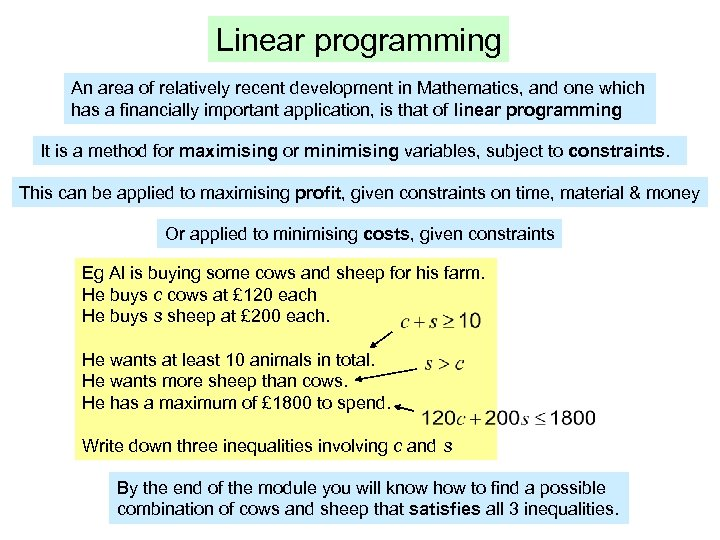Linear programming An area of relatively recent development in Mathematics, and one which has a financially important application, is that of linear programming It is a method for maximising or minimising variables, subject to constraints. This can be applied to maximising profit, given constraints on time, material & money Or applied to minimising costs, given constraints Eg Al is buying some cows and sheep for his farm. He buys c cows at £ 120 each He buys s sheep at £ 200 each. He wants at least 10 animals in total. He wants more sheep than cows. He has a maximum of £ 1800 to spend. Write down three inequalities involving c and s By the end of the module you will know how to find a possible combination of cows and sheep that satisfies all 3 inequalities.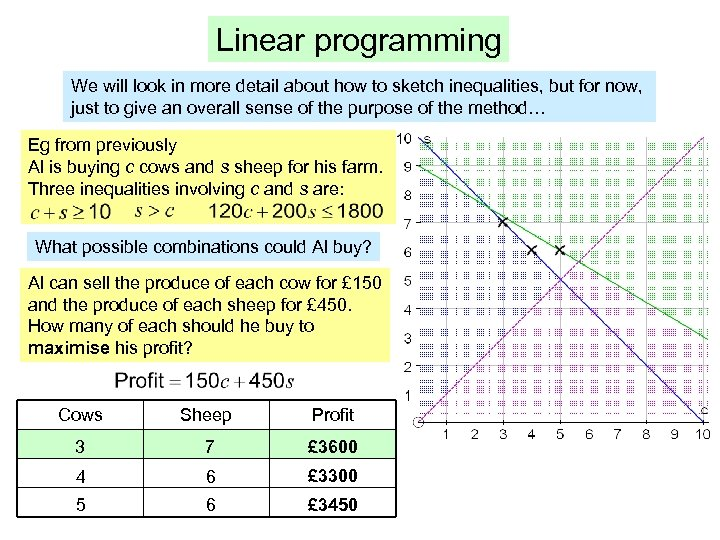Linear programming We will look in more detail about how to sketch inequalities, but for now, just to give an overall sense of the purpose of the method… Eg from previously Al is buying c cows and s sheep for his farm. Three inequalities involving c and s are: What possible combinations could Al buy? Al can sell the produce of each cow for £ 150 and the produce of each sheep for £ 450. How many of each should he buy to maximise his profit? Cows Sheep Profit 3 7 £ 3600 4 6 £ 3300 5 6 £ 3450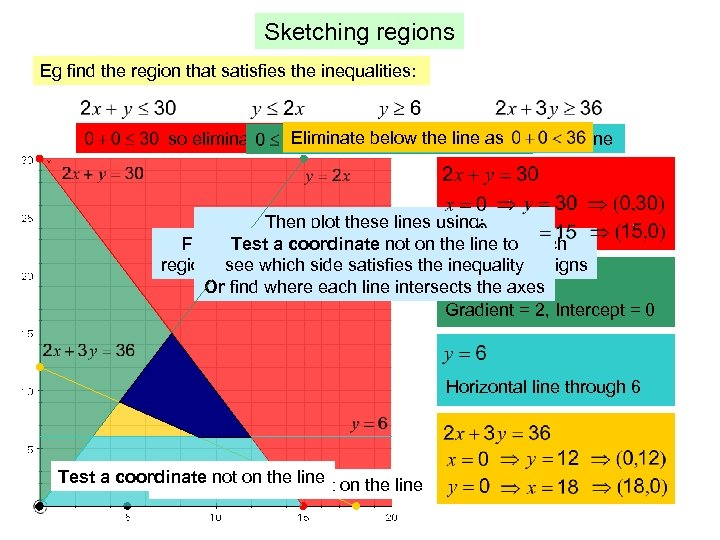Sketching regions Eg find the region that satisfies the inequalities: Eliminate below the line as so eliminate above the line If we want eliminate below the line so eliminate above the line Then plot these lines using: First identify the lines of the boundaries of each Test a coordinate not on the line to Either use intercept and gradient to plot region by replacing inequality signs with equals signs see which side satisfies the inequality Or find where each line intersects the axes Gradient = 2, Intercept = 0 Horizontal line through 6 Test a coordinate not on the line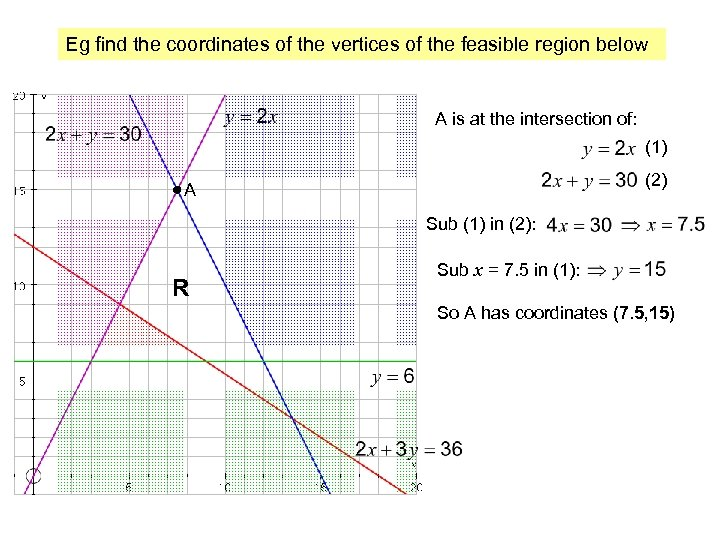Eg find the coordinates of the vertices of the feasible region below A is at the intersection of: (1) (2) A Sub (1) in (2): R Sub x = 7. 5 in (1): So A has coordinates (7. 5, 15)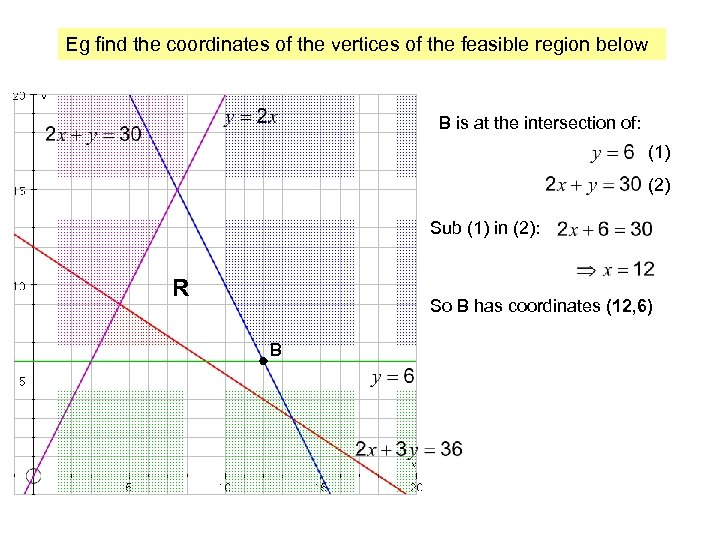Eg find the coordinates of the vertices of the feasible region below B is at the intersection of: (1) (2) Sub (1) in (2): R So B has coordinates (12, 6) B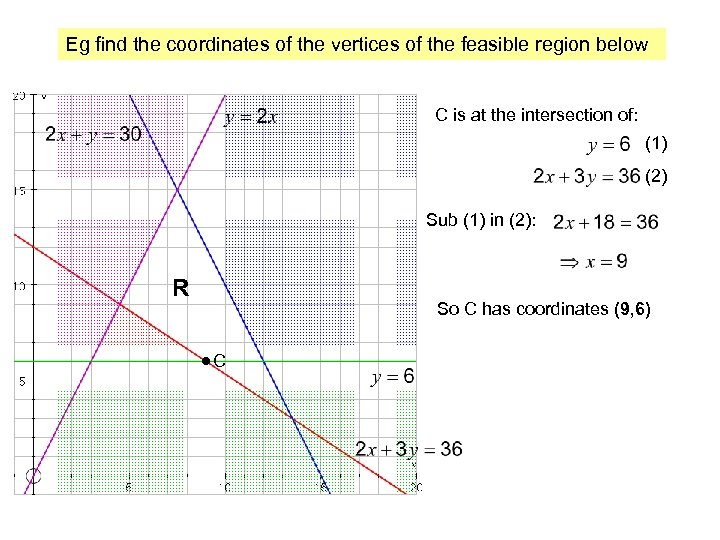Eg find the coordinates of the vertices of the feasible region below C is at the intersection of: (1) (2) Sub (1) in (2): R So C has coordinates (9, 6) C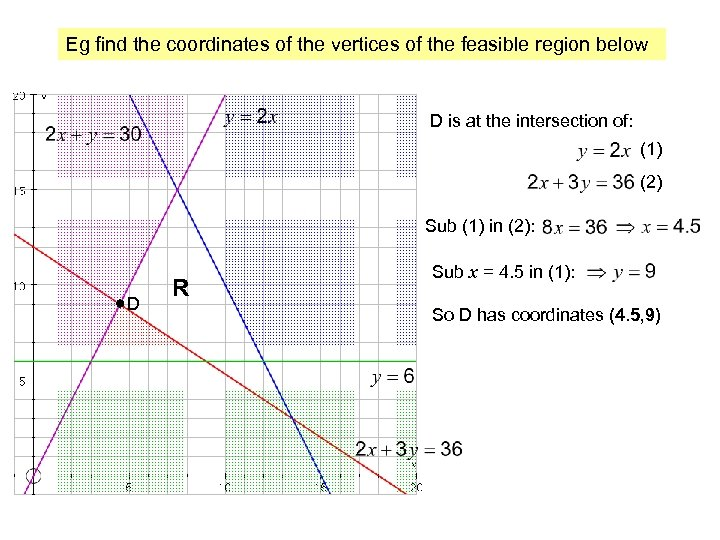Eg find the coordinates of the vertices of the feasible region below D is at the intersection of: (1) (2) Sub (1) in (2): D R Sub x = 4. 5 in (1): So D has coordinates (4. 5, 9)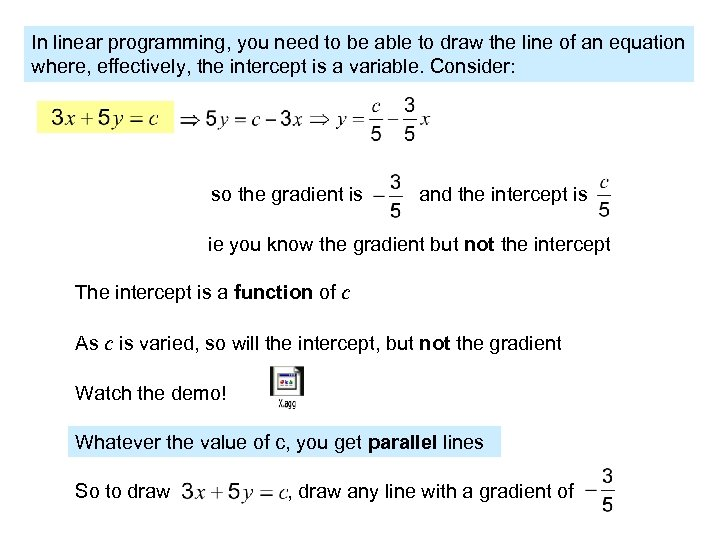In linear programming, you need to be able to draw the line of an equation where, effectively, the intercept is a variable. Consider: so the gradient is and the intercept is ie you know the gradient but not the intercept The intercept is a function of c As c is varied, so will the intercept, but not the gradient Watch the demo! Whatever the value of c, you get parallel lines So to draw , draw any line with a gradient of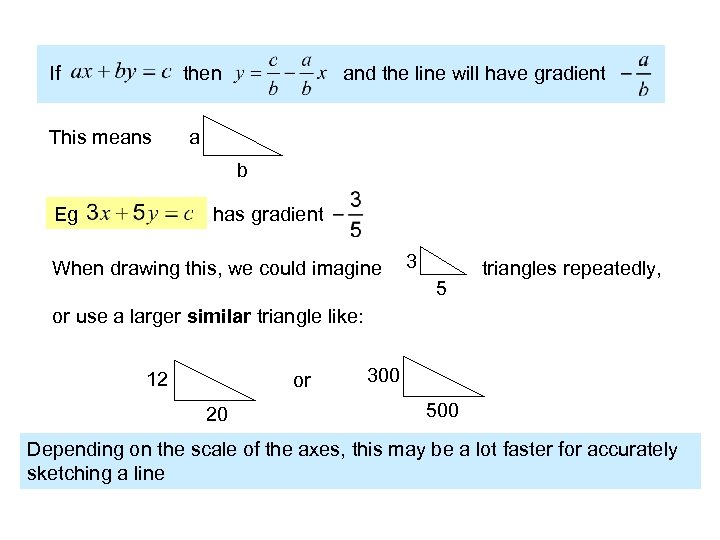If then This means and the line will have gradient a b Eg has gradient 3 When drawing this, we could imagine triangles repeatedly, 5 or use a larger similar triangle like: 12 or 20 300 500 Depending on the scale of the axes, this may be a lot faster for accurately sketching a line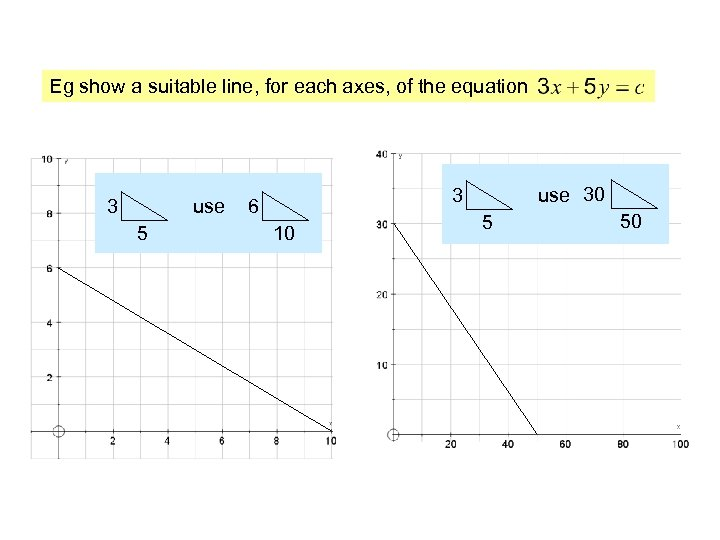Eg show a suitable line, for each axes, of the equation 3 use 5 use 30 3 6 10 5 50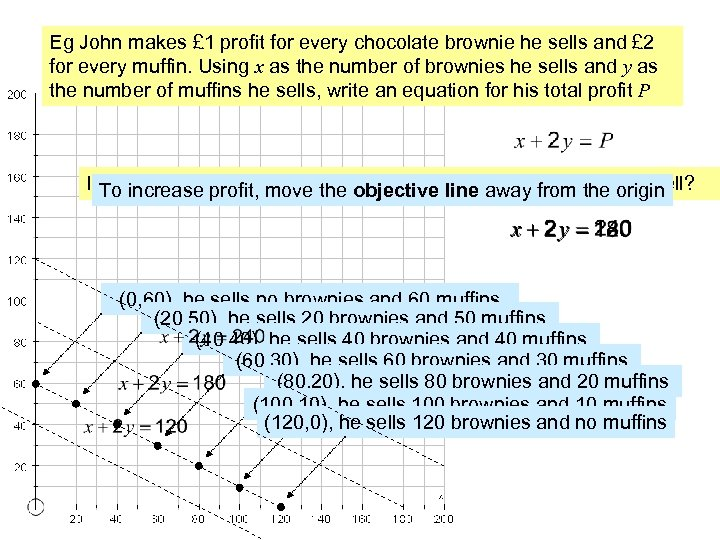Eg John makes £ 1 profit for every chocolate brownie he sells and £ 2 for every muffin. Using x as the number of brownies he sells and y as the number of muffins he sells, write an equation for his total profit P If he needs to make a profit of £ 240, what combination could he sell? If he needs to make a profit of £ 180, what combination could he sell? If he needs to make a profit of £ 120, what combination could he sell? To increase profit, move the objective line away from the origin (0, 60), he sells no brownies and 60 muffins (20, 50), he sells 20 brownies and 50 muffins (40, 40), he sells 40 brownies and 40 muffins (60, 30), he sells 60 brownies and 30 muffins (80, 20), he sells 80 brownies and 20 muffins (100, 10), he sells 100 brownies and 10 muffins (120, 0), he sells 120 brownies and no muffins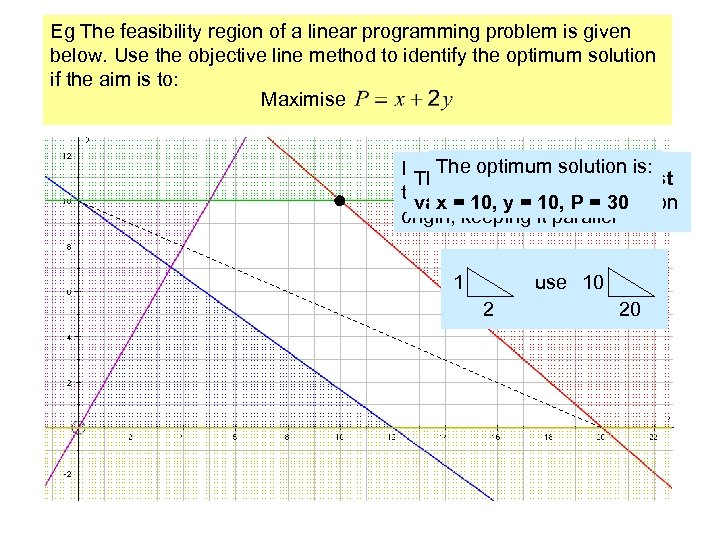Eg The feasibility region of a linear programming problem is given below. Use the objective line method to identify the optimum solution if the aim is to: Maximise The optimum solution is: Increase the profit by imagining Draw a ‘suitable’ line with The optimum point is the last the line sliding away from the correct gradient 30 value within the critical region x = 10, y = 10, P = origin, keeping it parallel 1 use 10 2 20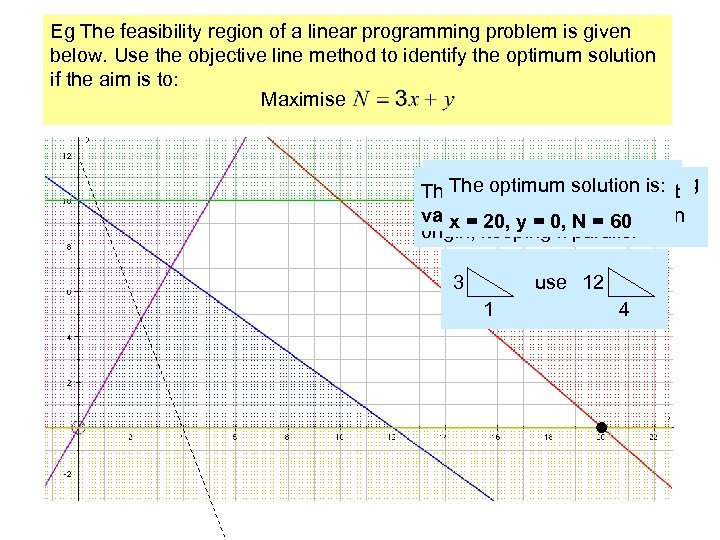Eg The feasibility region of a linear programming problem is given below. Use the objective line method to identify the optimum solution if the aim is to: Maximise Draw a ‘suitable’ line with Increase the profit by imagining The optimum solution is: The optimum point is the last the correct gradient the line sliding away from the value within the critical region x = 20, y = 0, N = 60 origin, keeping it parallel 3 use 12 1 4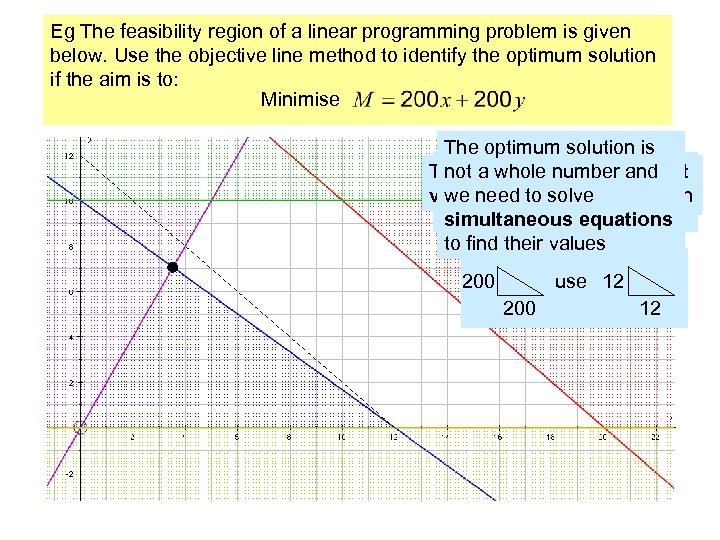Eg The feasibility region of a linear programming problem is given below. Use the objective line method to identify the optimum solution if the aim is to: Minimise The optimum solution is Minimise by imagining the The optimum point is the last not a whole number and Draw a ‘suitable’ line with line sliding towards the value within the critical region we need to solve the correct gradient origin, keeping it parallel simultaneous equations to find their values 200 use 12 200 12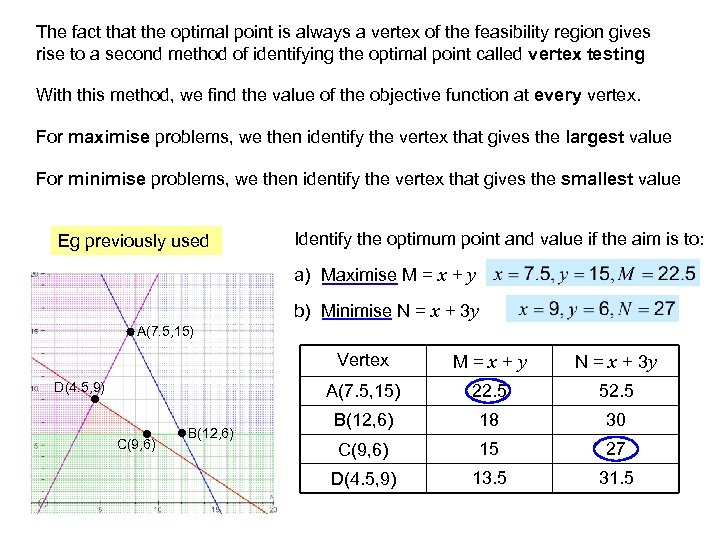The fact that the optimal point is always a vertex of the feasibility region gives rise to a second method of identifying the optimal point called vertex testing With this method, we find the value of the objective function at every vertex. For maximise problems, we then identify the vertex that gives the largest value For minimise problems, we then identify the vertex that gives the smallest value Eg previously used Identify the optimum point and value if the aim is to: a) Maximise M = x + y b) Minimise N = x + 3 y A(7. 5, 15) Vertex C(9, 6) B(12, 6) N = x + 3 y A(7. 5, 15) D(4. 5, 9) M = x + y 22. 5 52. 5 B(12, 6) 18 30 C(9, 6) 15 27 D(4. 5, 9) 13. 5 31. 5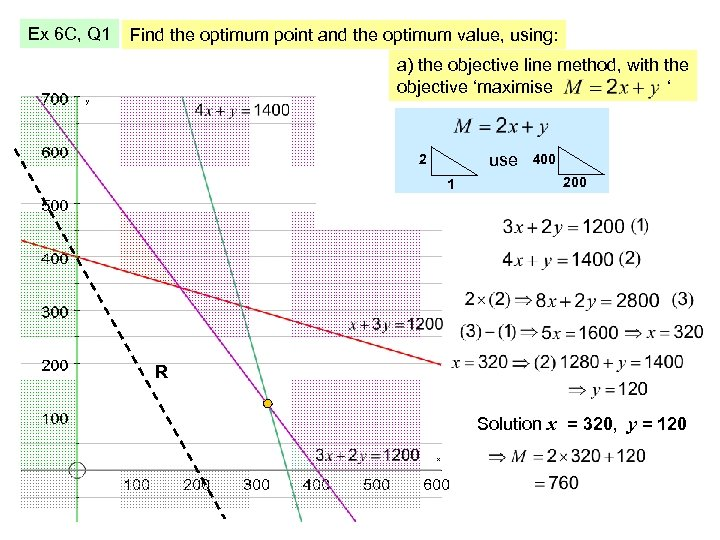Ex 6 C, Q 1 Find the optimum point and the optimum value, using: a) the objective line method, with the objective ‘maximise ‘ use 2 1 400 200 R Solution x = 320, y = 120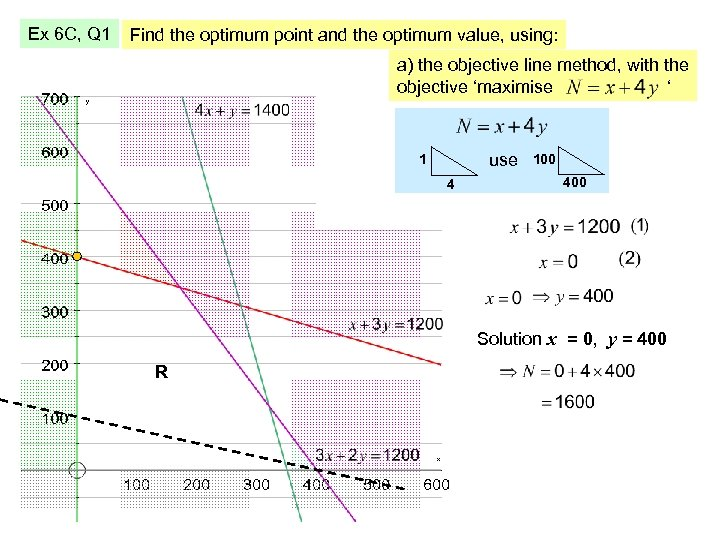Ex 6 C, Q 1 Find the optimum point and the optimum value, using: a) the objective line method, with the objective ‘maximise ‘ use 1 4 100 400 Solution x = 0, y = 400 R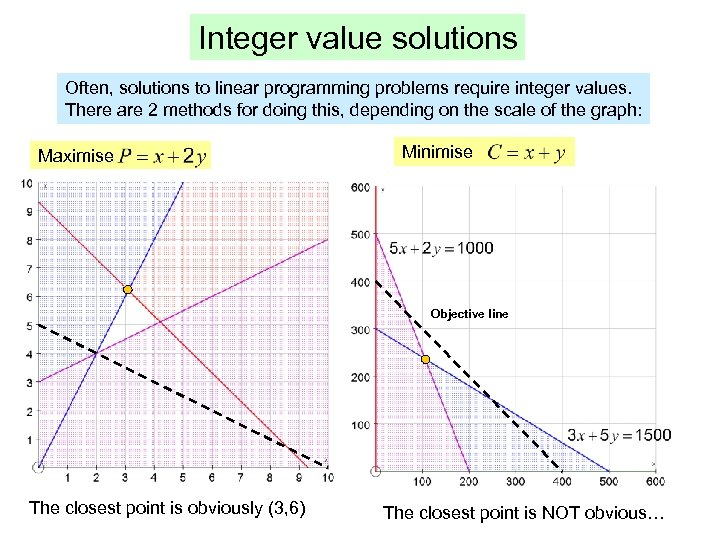Integer value solutions Often, solutions to linear programming problems require integer values. There are 2 methods for doing this, depending on the scale of the graph: Maximise Minimise Objective line The closest point is obviously (3, 6) The closest point is NOT obvious…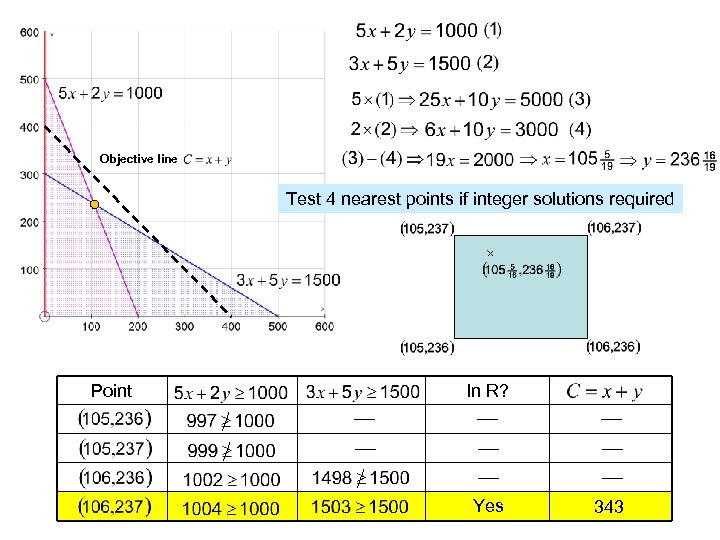Objective line Test 4 nearest points if integer solutions required Point In R? Yes 343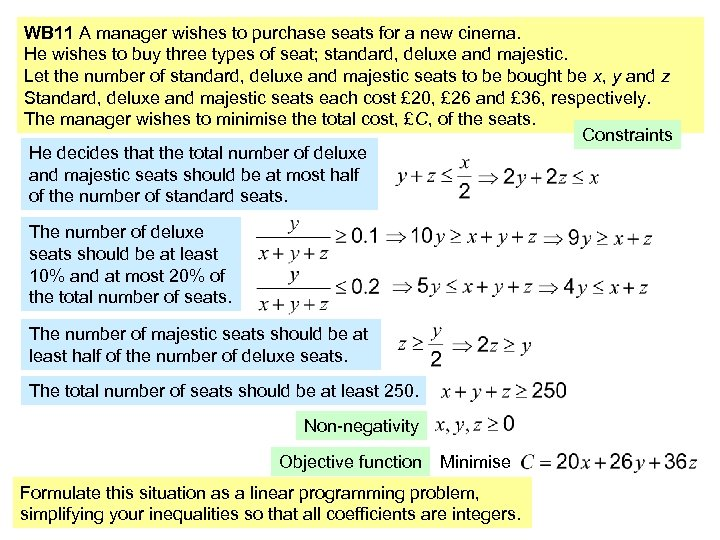WB 11 A manager wishes to purchase seats for a new cinema. He wishes to buy three types of seat; standard, deluxe and majestic. Let the number of standard, deluxe and majestic seats to be bought be x, y and z Standard, deluxe and majestic seats each cost £ 20, £ 26 and £ 36, respectively. The manager wishes to minimise the total cost, £C, of the seats. Constraints He decides that the total number of deluxe and majestic seats should be at most half of the number of standard seats. The number of deluxe seats should be at least 10% and at most 20% of the total number of seats. The number of majestic seats should be at least half of the number of deluxe seats. The total number of seats should be at least 250. Non-negativity Objective function Minimise Formulate this situation as a linear programming problem, simplifying your inequalities so that all coefficients are integers.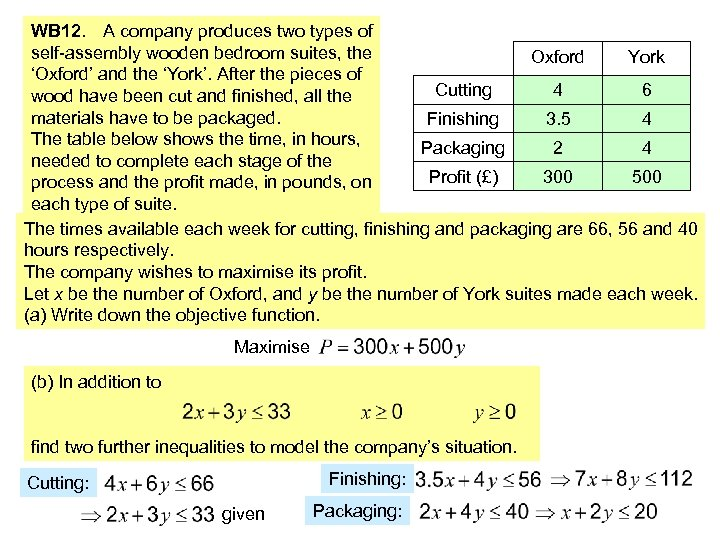WB 12. A company produces two types of self-assembly wooden bedroom suites, the Oxford York ‘Oxford’ and the ‘York’. After the pieces of Cutting 4 6 wood have been cut and finished, all the materials have to be packaged. Finishing 3. 5 4 The table below shows the time, in hours, Packaging 2 4 needed to complete each stage of the Profit (£) 300 500 process and the profit made, in pounds, on each type of suite. The times available each week for cutting, finishing and packaging are 66, 56 and 40 hours respectively. The company wishes to maximise its profit. Let x be the number of Oxford, and y be the number of York suites made each week. (a) Write down the objective function. Maximise (b) In addition to find two further inequalities to model the company’s situation. Finishing: Cutting: given Packaging: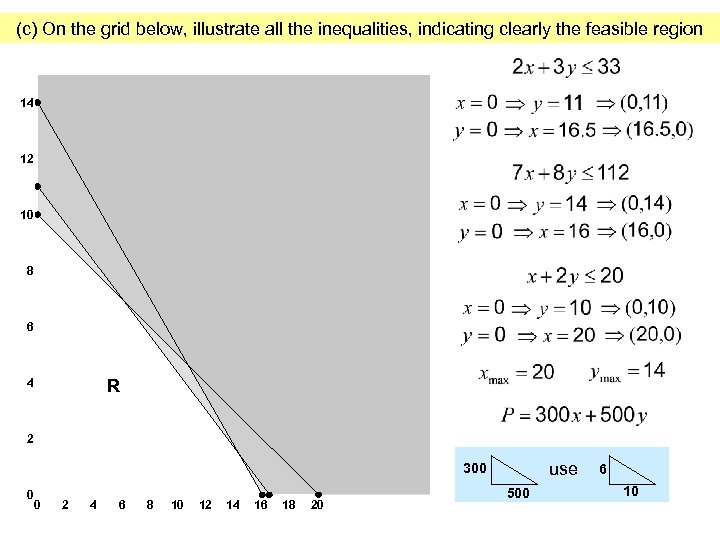(c) On the grid below, illustrate all the inequalities, indicating clearly the feasible region 14 12 10 8 6 R 4 2 use 300 0 0 2 4 6 8 10 12 14 16 18 20 500 6 10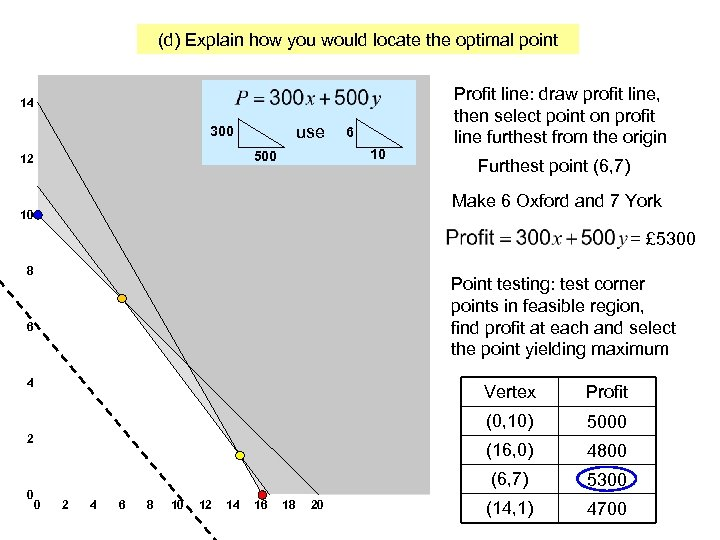(d) Explain how you would locate the optimal point 14 use 300 10 500 12 6 Profit line: draw profit line, then select point on profit line furthest from the origin Furthest point (6, 7) Make 6 Oxford and 7 York 10 = £ 5300 8 Point testing: test corner points in feasible region, find profit at each and select the point yielding maximum 6 4 Vertex (0, 10) 0 2 4 6 8 10 12 14 16 18 20 4800 (6, 7) 0 5000 (16, 0) 2 Profit 5300 (14, 1) 4700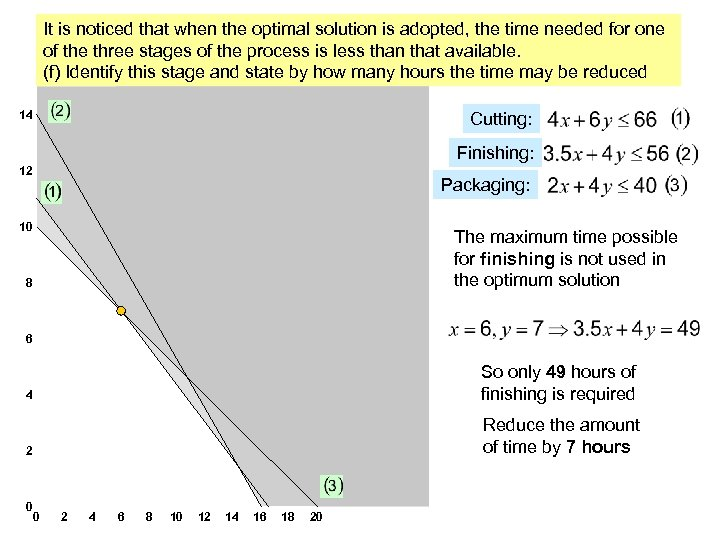It is noticed that when the optimal solution is adopted, the time needed for one of the three stages of the process is less than that available. (f) Identify this stage and state by how many hours the time may be reduced 14 Cutting: Finishing: 12 Packaging: 10 The maximum time possible for finishing is not used in the optimum solution 8 6 4 So only 49 hours of finishing is required 2 Reduce the amount of time by 7 hours 0 0 2 4 6 8 10 12 14 16 18 20